• matlab小波分析工具箱。利用小波分析工具箱实现相关算法以及matlab相关程序。
• Matlab小波分析工具箱原理与应用-Matlab小波分析工具箱原理与应用.part5.rar Matlab小波分析工具箱原理与应用
• Matlab小波分析工具箱原理与应用-Matlab小波分析工具箱原理与应用.part4.rar Matlab小波分析工具箱原理与应用
• Matlab小波分析工具箱原理与应用-Matlab小波分析工具箱原理与应用.part2.rar Matlab小波分析工具箱原理与应用
• Matlab小波分析工具箱原理与应用-Matlab小波分析工具箱原理与应用.part3.rar Matlab小波分析工具箱原理与应用
• Matlab小波分析工具箱原理与应用-Matlab小波分析工具箱原理与应用.part1.rar Matlab小波分析工具箱原理与应用
• matlab小波分析工具箱是一款非常实用的函数分析工具，它包含了图像化的工具和命令行函数，它可以实现测试、探索小波和小波包的特性，测试信号的统计特性和信号的组分，对一维信号执行连续小波变换等功能，需要的朋友...

matlab小波分析工具箱是一款非常实用的函数分析工具，它包含了图像化的工具和命令行函数，它可以实现测试、探索小波和小波包的特性，测试信号的统计特性和信号的组分，对一维信号执行连续小波变换等功能，需要的朋友快来本站下载吧!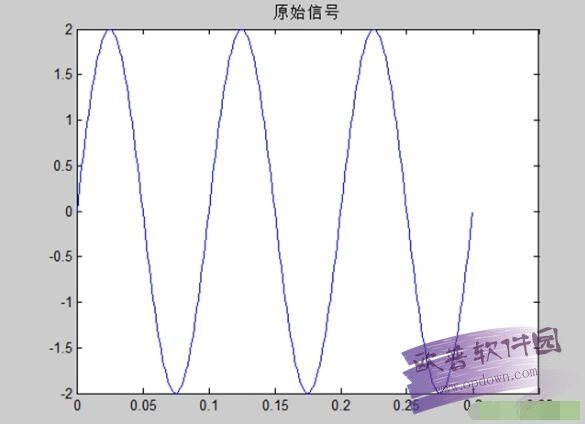matlab小波分析工具箱
功能说明
对一维、二维信号执行离散小波分析和综合
对一维、二维信号执行小波包分解(参见帮助Using Wavelet Packets)
对信号或图像进行压缩、去噪
测试、探索小波和小波包的特性
测试信号的统计特性和信号的组分
对一维信号执行连续小波变换
使用说明
运行install_emd.m文件可以实现此工具箱的安装，uninstall_emd.m实现卸载。
1、首先在本站下载emd工具箱，下载后解压放在matlab的work工作路径下package_emd文件夹。
2、打开matlab，选择File- Set Path- Add with Subfolders-你刚才下载的工具箱(package_emd)点进去- Save- Close。
3、此时选择work下package_emd文件夹作为工作路径，即是C:\Program Files\MATLAB\R2010a\toolbox\package_emd。
4、在Command Window里面输入mex -setup回车，问是否选择已有的编译器你选y回车，再问选择哪个编译器，你可以选择C++的那个选择相应的编号(如 2)回车，然后让你核对是否选择对了编译器等等，你输入y回车。就安装成功了
>> mex -setup
Would you like mex to locate installed compilers [y]/n? y
Select a compiler:
 Lcc-win32 C 2.4.1 in C:\PROGRA~1\MATLAB\R2010a\sys\lcc
 Microsoft Visual C++ 6.0 in C:\Program Files\Microsoft Visual Studio
 None
Compiler: 2
Compiler: Microsoft Visual C++ 6.0
Location: C:\Program Files\Microsoft Visual Studio
Are these correct [y]/n? y
Trying to update options file: C:\Users\Administrator\AppData\Roaming\MathWorks\MATLAB\R2010a\mexopts.bat
From template: C:\PROGRA~1\MATLAB\R2010a\bin\win32\mexopts\msvc60opts.bat
Done . . .
Warning: The MATLAB C and Fortran API has changed to support MATLAB
variables with more than 2^32-1 elements. In the near future
you will be required to update your code to utilize the new
http://www.mathworks.com/support/solutions/en/data/1-5C27B9/?solution=1-5C27B9
Building with the -largeArrayDims option enables the new API.
MATLAB的emd的工具箱 可是不知道怎么用 也不知道怎么用它来处理txt中的数据

展开全文• Matlab小波分析工具箱原理与应用，无水印完整版，分享给大家
• 数据建模Matlab小波分析工具箱原理与应用资料.rar
• matlab小波分析工具箱原理与应用 清晰小容量版 比较基础
MATLAB小波分析工具箱的使用
1.在命令窗口输入：wavemenu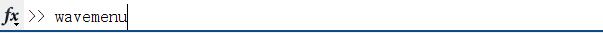1.弹出窗口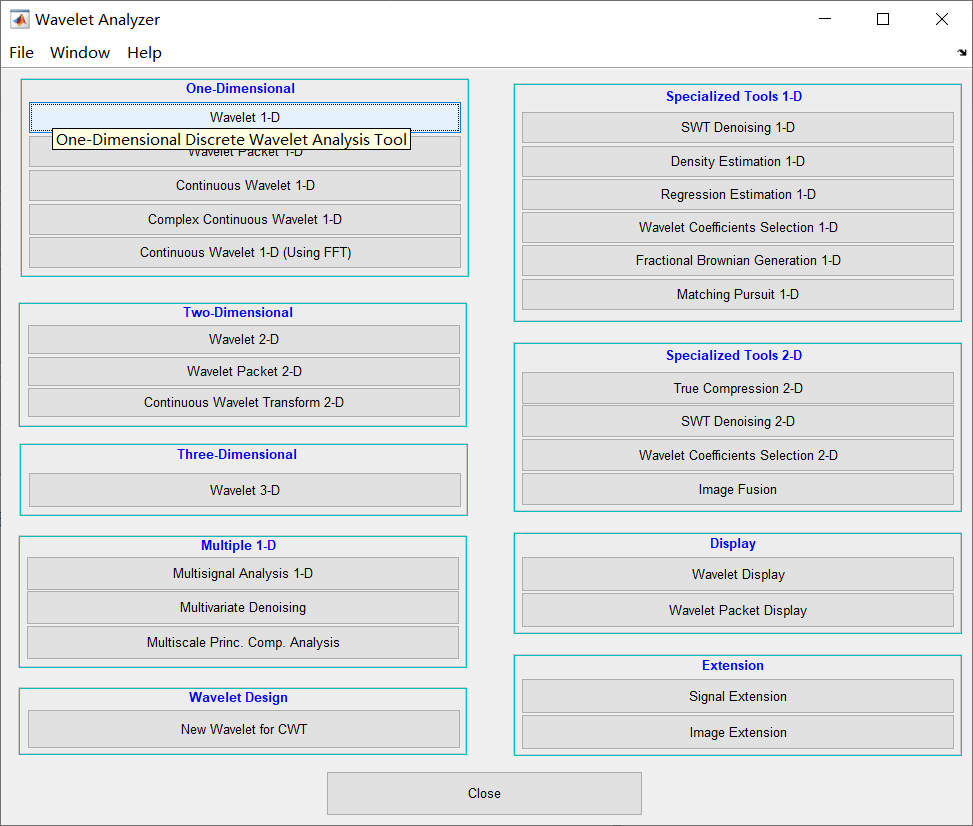点击需要的工具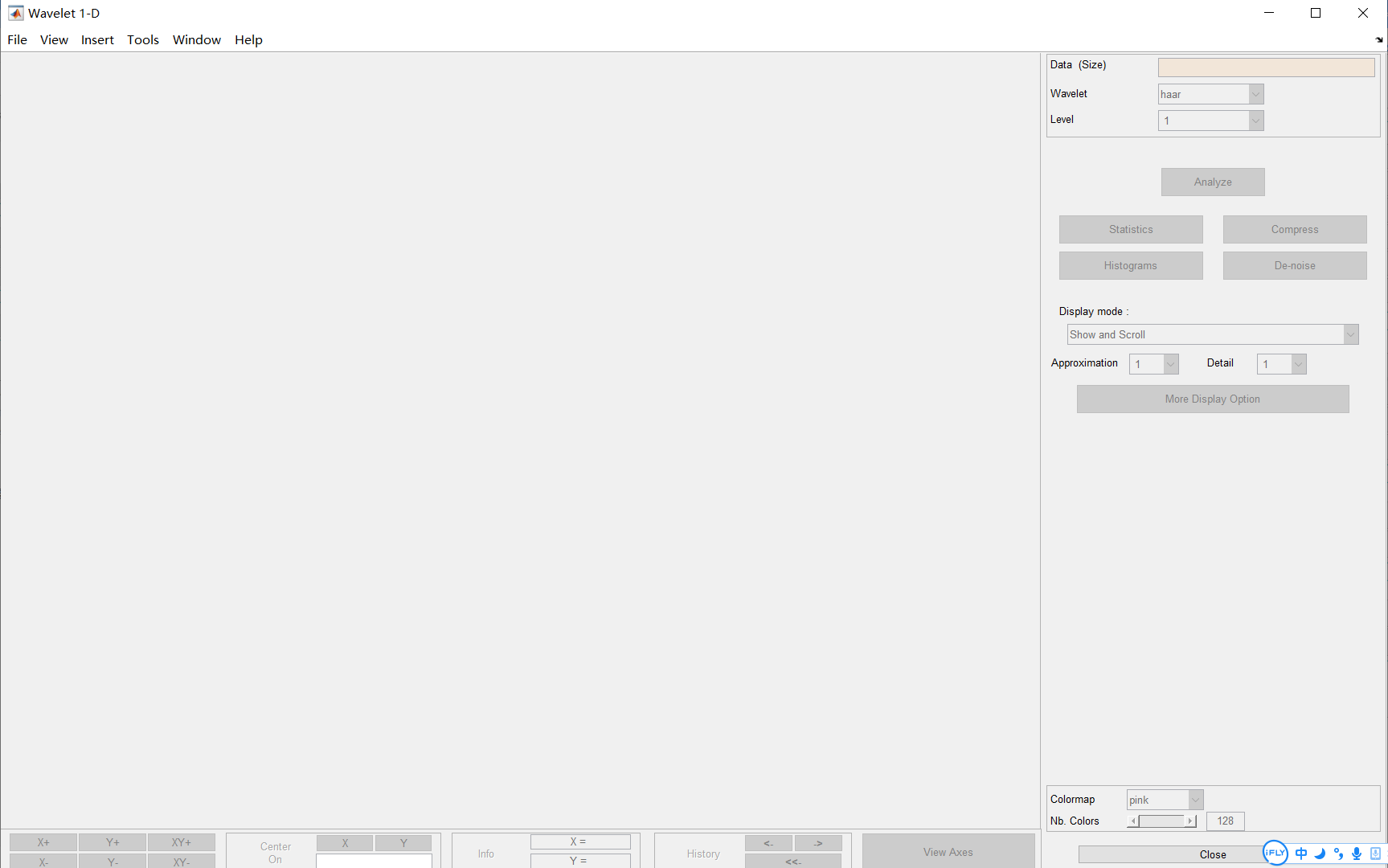加载数据：flie——>load——>signal 进入自己数据文件夹，点击需要分析的数据。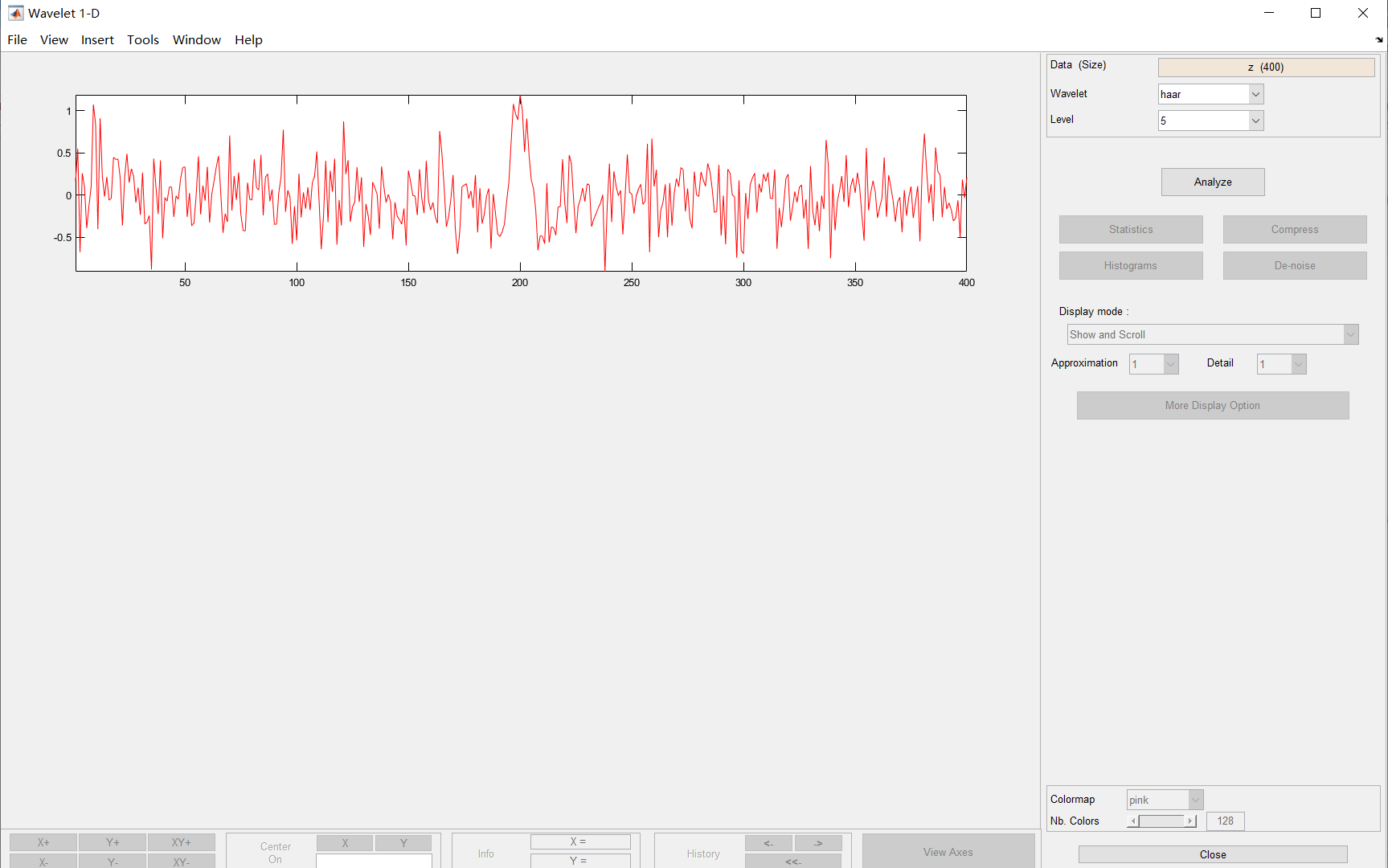4.右边框1展示数据名称和长度；框2小波类型，有的小波有不同的版本；框4小波分解级数。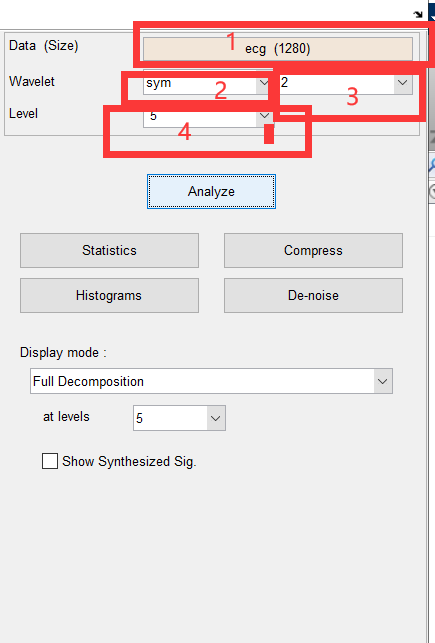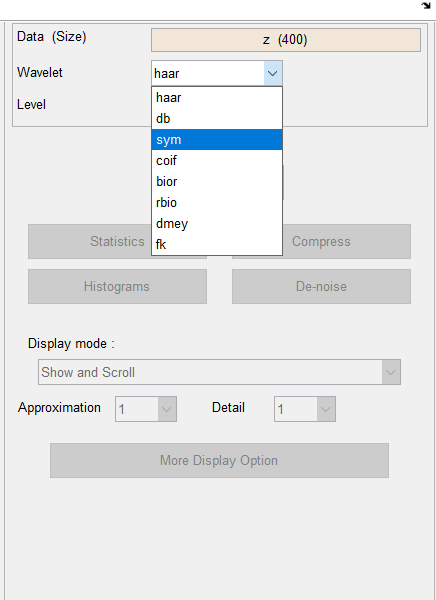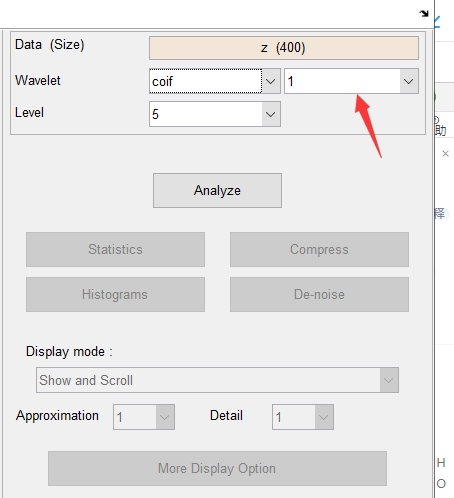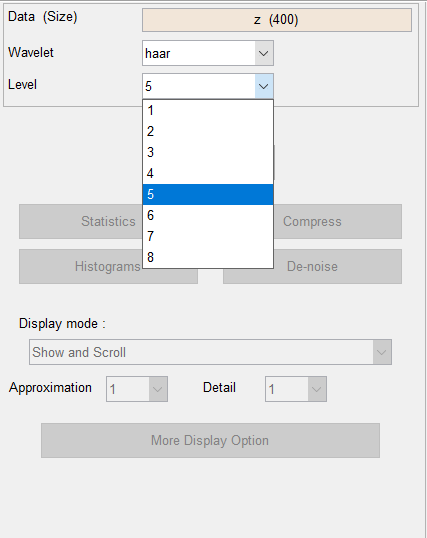4. 设置好右边参数后，点击Analyze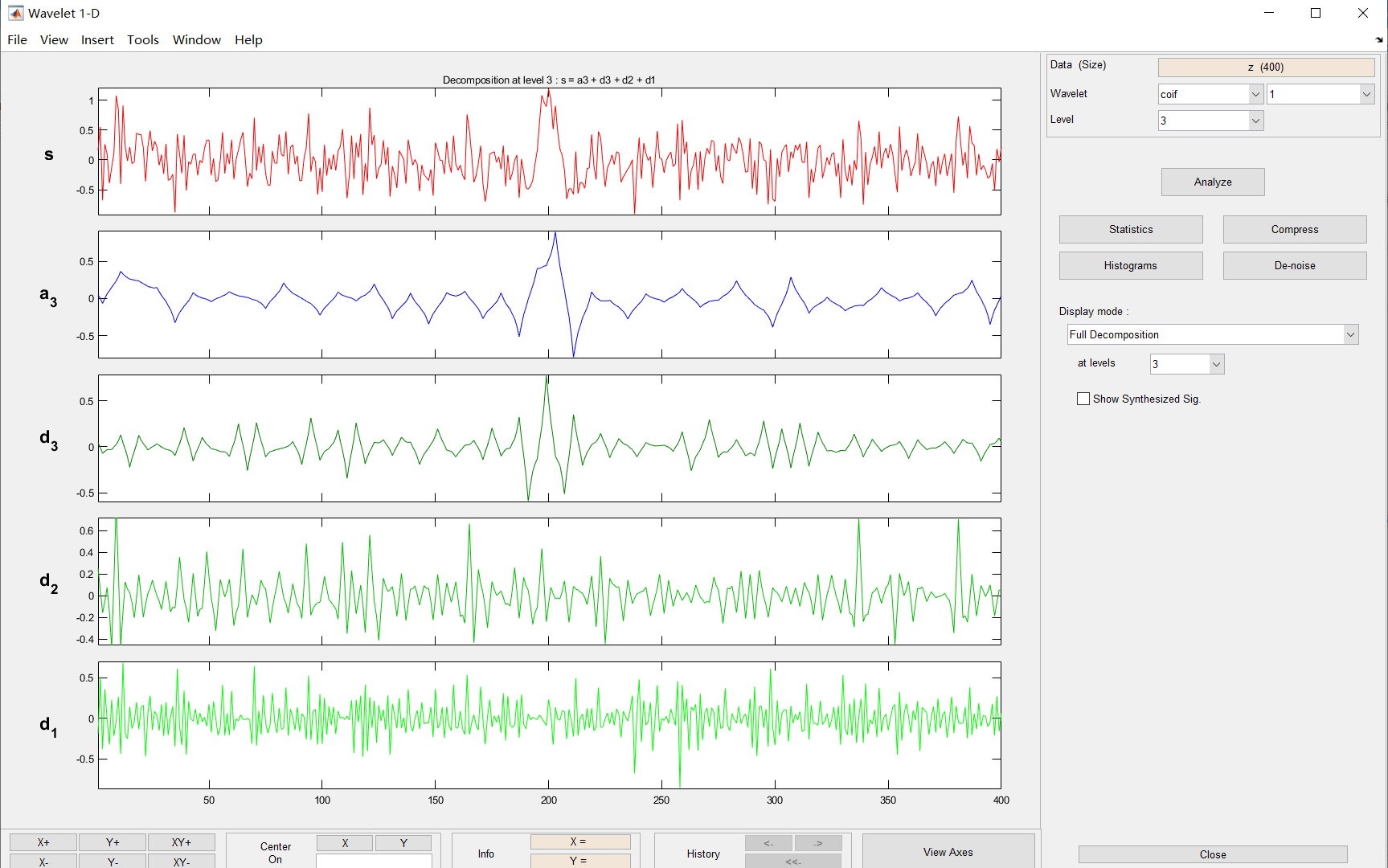展开全文• 本人倾情奉献，有关小波理论的《Matlab小波分析工具箱原理与应用》一本好书，对初学和进阶者均有一定的帮助。
• 该书籍十分通熟易懂，并且有matlab案例，非常时候入门或者进阶。可以发现其中的精彩之处，与《小波十讲》搭配研究，更可以加深理论学习，matlab编程。（小波十讲我也上传了）。版权归书籍作者所有。
• matlab小波分析工具箱常用函数  1.Cwt :一维连续小波变换 格式：coefs=cwt(s,scales,'wavename')  coefs=cwt(s,scales,'wavename','plot') scales:尺度向量，可以为离散值，表示为[a1,a2,a3……]，...


matlab小波分析工具箱常用函数

1.Cwt :一维连续小波变换
格式：coefs=cwt(s,scales,'wavename')
coefs=cwt(s,scales,'wavename','plot')
scales:尺度向量，可以为离散值，表示为[a1,a2,a3……]，也可为连续值，表示为[amin:step:amax]
2.dwt:单尺度一维离散小波变换
格式：[ca,cd]=dwt(x,'wavename') %使用小波'wname'对信号X进行单层分解，求得的近似系数存放在数组cA中，细节系数存放在数组cD中
[ca,cd]=dwt(x,lo-d,hi-d)
先利用小波滤波器指令wfilters求取分解用低通滤波器lo-d和高通滤波器hi-d。
[lo-d,hi-d]=wfilters('haar','d');
[ca,cd]=dwt(s,lo-d,hi-d)

DWT2是二维单尺度小波变换，其可以通过指定小波或者分解滤波器进行二维单尺度小波分解   DWT2的一种语法格式是[cA,cH,cV,cD]=dwt2(X,'wname')
WAVEDEC2是二维多尺度小波分解   WAVEDEC2的语法格式是[C,S]=wavedec2(X,N,'wname')，其中N为大于1的正整数
也就是说DWT2只能对某个输入矩阵X进行一层分解，而WAVEDEC2可以对输入矩阵X进行N层分解

3.idwt:单尺度一维离散小波逆变换
4.wfilters
格式：[lo-d,hi-d,lo-r,hi-r]=wfilters('wname')
[f1,f2]=wfilters('wname','type')
type=d(分解滤波器)、R(重构滤波器)、l(低通滤波器)、h(高通滤波器)
5.dwtmode 离散小波变换模式
格式：dwtmode
dwtmode('mode')
mode:zdp补零模式，sym对称延拓模式，spd平滑模式
6.wavedec多尺度一维小波分解
格式：[c,l]=wavedec(x,n,'wname')%利用小波'wname'对信号X进行多层分解,n:尺度。
[c,l]=wavedec(x,n,lo-d,hi-d)
[C,L]=wavedec(X,1,’wname’)中返回的近似和细节都存放在C中，即C=[cA,cD]，L存放是近似和各阶细节系数对应的长度
7.appcoef 提取一维小波变换低频系数
格式：A=appcoef(c,l,'wavename',N)；%  利用小波'wname'从分解系数[c,l]中提取第N层近似系数
A=appcoef(c,l,lo-d,hi-d,N)  N是尺度，可省略
例：
s=leleccum(1:2000)
subplot(421)
plot(s);
title('原始信号')
[c,l]=wavedec(s,3,'db1');
ca1=appcoef(c,l,'db1',1);
subplot(445)
plot(ca1);
ylabel('ca1');
ca2=appcoef(c,l,'db1',2);
subplot(4,8,17)
plot(ca2);
ylabel('ca2');
8.detcoef  提取一维小波变换高频系数
格式：d=detcoef(c,l,N),N尺度的高频系数
d=detcoef(c,l,) 最后一尺度的高频系数
例：
s=leleccum(1:2000)
subplot(421)
plot(s);
title('原始信号')
[c,l]=wavedec(s,3,'db1');
cd1=detcoef(c,l,1);
subplot(445)
plot(cd1);
ylabel('cd1');
cd2=detcoef(c,l,2);
subplot(4,8,17)
plot(cd2);
ylabel('cd2');
9.waverec 多尺度一维小波重构
格式：x=waverec(c,l,'wavename')
x=waverec(c,l,lo-r,hi-r)
x=waverec(waverec(c,l,'wavename'),'wavename')
10.upwlev 单尺度一维小波的重构
格式：[nc,na,ca]=upwlev(c,l,'wname')
[nc,na,ca]=upwlev(c,l,lo-r,hi-r)
返回上一尺度的分解结构并提取最后一尺度的低频分量，等价于[c,l]=wavedec(x,N-1，'wavename')
11.wrcoef 对一维小波系数进行单支重构
格式：x=wrcoef('type',c,l,'wavename',N)
x=wrcoef('type',c,l,'wavename')
x=wrcoef('type',c,l,lo-r,hi-r,N)
x=wrcoef('type',c,l,lo-r,hi-r)

12.upcoef一维系数的直接小波重构

格式：y=wrcoef('o',x,'wavename',N,L)

y=wrcoef('o',x,'wavename',N)

y=wrcoef('o',x,lo-r,hi-r,N,L)

用来计算向量X(信号系数)向上N步的重构小波系数，N为正整数。O=a低频重构，d高频重构，L是对向量中间长度L进行重构。

13.wpdec 一维小波包分解

格式：T=wpdec(X,N,'wavename',E,P)

14.wprec  一维小波包重构

格式：X=wpdec(T)

15.wpcoef 计算小波系数
格式：X=wpdec(t,n)

X=wpdec(t)

16.wprcoef 小波包分解系数的重构，一维或二维小波包分析函数，每次只能对一个节点重构。多个节点可重复调用来实现

格式：X=wprdec(t,n)

X=wprdec(t)

17.wpfun 小波包函数

格式：[wpms,x]=wpfun('wname',num,prec)

18.wpsplt 分解小波包

格式：t=wpsplt(t,n)

[t,ca,cd]=wpsplt(t,n)

[t,ca,ch,cv,cd,]=wpsplt(t,n)

19.wpjoin 重新组合小波包

格式：t=wpjoin(t,n)

[t,x]=wpjoin(t,n)
[t,x]=wpjoin(t)

20.wpcutree 剪切小波包分解树
格式：t=wpcutree(t,L)  L层对t树剪切
21.besttree  计算最佳树
格式：t=besttree(t)
[T,E]=besttree(t)
[T,E,N]=besttree(t)
22.bestlevt  计算完整最佳小波包树
格式：t=bestlevt(t)
[T,E]=bestlevt(t)
23.wp2wtree 从小波包树中提取小波树
格式：t=wp2wtree(t)
转自：http://blog.csdn.net/baijingjing425/article/details/7671870

展开全文• 小波分析工具箱使用（一）   .wiz-todo, .wiz-todo-img {width: 16px; height: 16px; cursor: default; padding: 0 10px 0 2px; vertical-align: -10%;-webkit-user-select: none;} .wiz-todo-label { display: ...
<!DOCTYPE html>
<meta http-equiv="Content-Type" content="text/html; charset=unicode">
<title>小波分析工具箱使用（一）</title>
<!--mark |wiz_custom_css| for wizeditor replace it-->
<style type="text/css" id="wiz_todo_style_id" wiz_link_version="01.00.09">.wiz-todo, .wiz-todo-img {width: 16px; height: 16px; cursor: default; padding: 0 10px 0 2px; vertical-align: -10%;-webkit-user-select: none;} .wiz-todo-label { display: inline-block; padding-top: 7px; padding-bottom: 6px; line-height: 1.5;} .wiz-todo-label-checked {  color: #666;} .wiz-todo-label-unchecked {text-decoration: initial;} .wiz-todo-completed-info {padding-left: 44px; display: inline-block; } .wiz-todo-avatar { width:20px; height: 20px; vertical-align: -20%; margin-right:10px; border-radius: 2px;} .wiz-todo-account, .wiz-todo-dt { color: #666; }</style><
展开全文• 酝葬贼造葬遭应用丛书摇董长虹 主编摇 摇高志 余啸海 编著·北京 ·摇图书在版编目(悦陨孕)数据摇酝葬贼造葬遭 辕 ，小波分析工具箱原理与应用 高志 余啸海编援— ： ，圆园园源援员著 北京 国防工业出版社摇(...
• 1.Cwt :一维连续小波变换 格式：coefs=cwt(s,scales,'wavename')  coefs=cwt(s,scales,'wavename','plot') ...scales:尺度向量，可以为离散值，表示为[a1,a2,a3……]，也可为连续值，表示为[amin:step:amax] ...
• 格式：T=wpdec(X,N,'wavename',E,P) 14.wprec 一维小波包重构 格式：X=wpdec(T) 15.wpcoef 计算小波系数 格式：X=wpdec(t,n) X=wpdec(t) 16.wprcoef 小波包分解系数的重构，一维或二维小波包分析函数，每次只能对一......

# matlab小波分析工具箱matlab 订阅